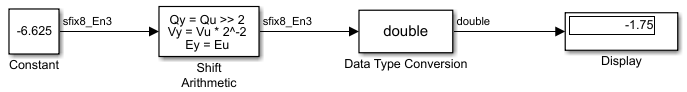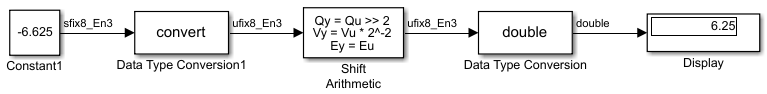## Shifts

Nearly all microprocessors and digital signal processors support well-defined bit-shift (or simply shift) operations for integers. For example, consider the 8-bit unsigned integer 00110101. The results of a 2-bit shift to the left and a 2-bit shift to the right are shown in the following table.

Shift OperationBinary ValueDecimal Value

No shift (original number)

00110101

53

Shift left by 2 bits

11010100

212

Shift right by 2 bits

00001101

13

You can perform a shift using the Simulink® Shift Arithmetic block. Use this block to perform a bit shift, a binary point shift, or both

### Shifting Bits to the Right

The special case of shifting bits to the right requires consideration of the treatment of the leftmost bit, which can contain sign information. A shift to the right can be classified either as a logical shift right or an arithmetic shift right. For a logical shift right, a 0 is incorporated into the most significant bit for each bit shift. For an arithmetic shift right, the most significant bit is recycled for each bit shift.

The Shift Arithmetic block performs an arithmetic shift right and, therefore, recycles the most significant bit for each bit shift right. For example, given the fixed-point number 11001.011 (-6.625), a bit shift two places to the right with the binary point unmoved yields the number 11110.010 (-1.75), as shown in the model below:To perform a logical shift right on a signed number using the Shift Arithmetic block, use the Data Type Conversion block to cast the number as an unsigned number of equivalent length and scaling, as shown in the following model. The model shows that the fixed-point signed number 11001.001 (-6.625) becomes 00110.010 (6.25).## SupportGet trial now